##### Representing Graphs

We define a graph to be a set of vertices with a set of pairs of these vertices called edges. The representation of graphs takes on different requirements depending upon whether the intended consumer is a person or a machine. Computers digest graphs best as data structures such as adjacency matrices or lists. People prefer a visualization of the structure as a collection of points connected by lines, which implies adding geometric information to the graph.

In the complete graph on five vertices, denoted $K_5$, each vertex is adjacent to all other vertices. CompleteGraph[n] constructs the complete graph on $n$ vertices.

`ShowGraph[ CompleteGraph ];`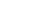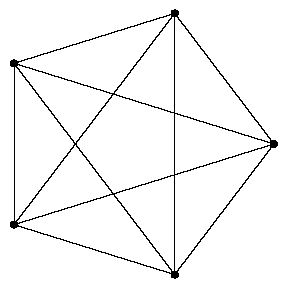The adjacency matrix of $K_5$ shows that each vertex is adjacent to all other vertices. The main diagonal consists of zeros, since there are no selfŁloops in the complete graph, meaning edges from a vertex to itself.

`TableForm[ Edges[CompleteGraph] ]`
`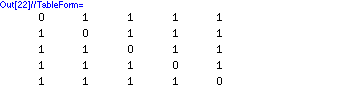`

The standard embedding of $K_5$ consists of five vertices equally spaced on a circle.

`Vertices[ CompleteGraph ]`
`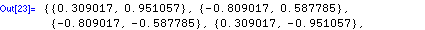`

The number of vertices in a graph is termed the order of the graph.

`V[ CompleteGraph ]`
`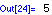`

With an optional argument to specify whether we count directed or undirected edges, M returns the number of edges in a graph.

`{M[CompleteGraph], M[CompleteGraph, Directed]}`
`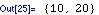`

A star is a tree with one vertex of degree $n - 1$. Adding any new edge to a star produces a cycle of length 3.

`ShowGraph[ AddEdge[Star, {1,2}] ];`The adjacency list representation of a graph consists of $n$ lists, one list for each vertex $v_i$, $1 <= i <= n$, which records the vertices $v_i$ is adjacent to. Each vertex in the complete graph is adjacent to all other vertices.

`TableForm[ ToAdjacencyLists[CompleteGraph] ]`
`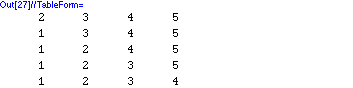`

There are $n \left(n - 1\right)$ ordered pairs of edges defined by a complete graph of order $n$.

`ToOrderedPairs[ CompleteGraph ]`
`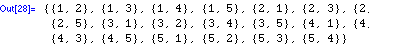`

An induced subgraph of a graph $G$ is a subset of the vertices of $G$ together with any edges whose endpoints are both in this subset. An induced subgraph that is complete is called a clique. Any subset of the vertices in a complete graph defines a clique.

`ShowGraph[ InduceSubgraph[CompleteGraph, RandomSubset[Range]] ];`The vertices of a bipartite graph have the property that they can be partitioned into two sets such that no edge connects two vertices of the same set. Contracting an edge in a bipartite graph can ruin its bipartiteness.

`ShowGraph[ Contract[ CompleteGraph[6,6],{1,7} ] ];`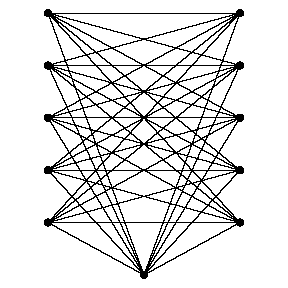A breadthŁfirst search of a graph explores all the vertices adjacent to the current vertex before moving on. A breadthŁfirst traversal of a simple cycle alternates sides as it wraps around the cycle.

`BreadthFirstTraversal[Cycle,1]`
`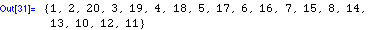`

In a depthŁfirst search, the children of the first son of a vertex are explored before visiting his brothers. The depthŁfirst traversal differs from the breadthŁfirst traversal above, proceeding directly around the cycle.

`DepthFirstTraversal[Cycle, 1]`
`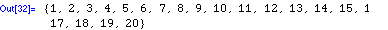`

Different drawings or embeddings of a graph can reveal different aspects of its structure. The default embedding for a grid graph is a ranked embedding from all the vertices on one side. Ranking from the center vertex yields a different but interesting drawing.

`ShowGraph[ RankedEmbedding[GridGraph[5,5],{13}]];`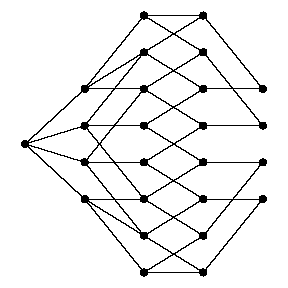The radial embedding of a tree is guaranteed to be planar, but radial embeddings can be used with any graph. Here we see a radial embedding of a random labeled tree.

`ShowGraph[ RandomTree ];`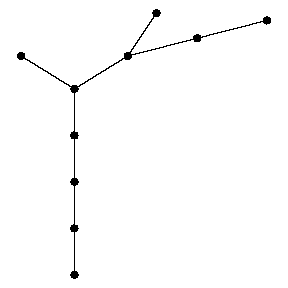An interesting general heuristic for drawing graphs models the graph as a system of springs and lets Hooke's law space the vertices. Here it does a good job illustrating the join operation, where each vertex of $K_7$ is connected to each of two disconnected vertices. In achieving the minimum energy configuration, these two vertices end up on different sides of $K_7$.

`ShowGraph[ SpringEmbedding[ GraphJoin[EmptyGraph, CompleteGraph]]];`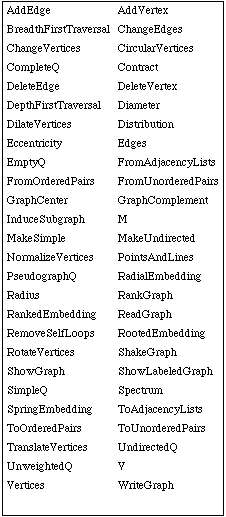Combinatorica functions for representing graphs.